# IFERROR with VLOOKUP in Excel - elegant way to trap errors

In this tutorial, we will be looking at how to use IFERROR and VLOOKUP functions together to trap and handle different errors. In addition, you are going to learn how to do sequential vlookups in Excel by nesting multiple IFERROR functions one onto another.

Excel VLOOKUP and IFERROR - these two functions may be pretty hard to understand separately, let alone when they are combined. In this article, you will find a few easy-to-follow examples that address common uses and clearly illustrate the formulas' logic.

If you don't have much experience with IFERROR and VLOOKUP functions, it may be a good idea to revise their basics first by following the above links.

## IFERROR VLOOKUP formula to handle #N/A and other errors

When Excel Vlookup fails to find a lookup value, it throws an #N/A error, like this: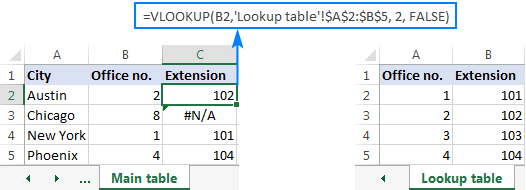Depending on your business needs, you may want to disguise the error with your own text, zero, or a blank cell.

### Example 1. IFERROR with VLOOKUP formula to replace errors with your own text

If you'd like to replace the standard error notation with your custom text, wrap your VLOOKUP formula in IFERROR, and type any text you want in the 2nd argument (value_if_error), for example "Not found":

With the lookup value in B2 in the Main table and the lookup range A2:B4 in the Lookup table, the formula takes the following shape:

`=IFERROR(VLOOKUP(B2,'Lookup table'!\$A\$2:\$B\$5, 2, FALSE), "Not found") `

The screenshot below shows our Excel IFERROR VLOOKUP formula in action: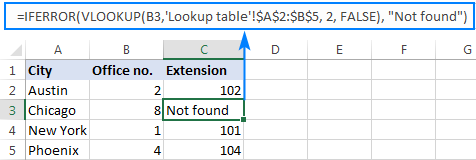The result looks much more understandable and far less intimidating, isn't it?

In a similar manner, you can use INDEX MATCH together with IFERROR:

`=IFERROR(INDEX('Lookup table'!\$B\$2:\$B\$5,MATCH(B2,'Lookup table'!\$A\$2:\$A\$5,0)), "Not found")`

The IFERROR INDEX MATCH formula is especially useful when you want to pull values from a column that lies to the left of the lookup column (left lookup), and return your own text when nothing is found.

### Example 2. IFERROR with VLOOKUP to return blank or 0 if nothing is found

If you don't want to show anything when the lookup value is not found, have IFERROR display an empty string (""):

IFERROR(VLOOKUP(),"")

In our example, the formula goes as follows:

`=IFERROR(VLOOKUP(B2,'Lookup table'!\$A\$2:\$B\$5, 2, FALSE), "") `

As you can see, it returns nothing when the lookup value is not in the search list.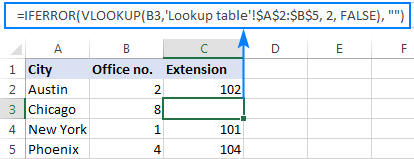If you'd like to replace the error with the zero value, put 0 in the last argument:

`=IFERROR(VLOOKUP(B2,'Lookup table'!\$A\$2:\$B\$5, 2, FALSE), 0)`

Word of caution! Excel IFERROR function catches all kinds of errors, not only #N/A. Is it good or bad? All depends on your goal. If you want to mask all possible errors, IFERROR Vlookup is the way to go. But it may be an unwise technique in many situations.

For example, if you've created a named range for your table data, and misspelled that name in your Vlookup formula, IFERROR will catch a #NAME? error and replace it with "Not found" or any other text you supply. As the result, you may never know your formula is delivering wrong results unless you spot the typo yourself. In such a case, a more reasonable approach would be trapping only #N/A errors. For this, use IFNA Vlookup formula in Excel 2013 and higher, IF ISNA VLOOKUP in all Excel versions.

The bottom line is: be very careful when choosing a companion for your VLOOKUP formula :)

## Nest IFERROR within VLOOKUP to always find something

Imagine the following situation: you look up a specific value in a list and do not find it. What choices do you have? Either get an N/A error or show your own message. Actually, there is a third option - if your primary vlookup stumbles, then search for something else that is definitely there!

Taking our example further, let's create some sort of dashboard for our users that will show them an extension number of a specific office. Something like this: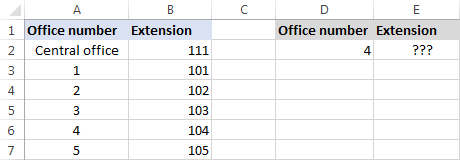So, how do you pull the extension from column B based on the office number in D2? With this regular Vlookup formula:

`=VLOOKUP(\$D\$2,\$A\$2:\$B\$7,2,FALSE)`

And it will work nicely as long as your users enter a valid number in D2. But what if a user inputs some number that does not exist? In this case, let them call the central office! For this, you embed the above formula in the value argument of IFERROR, and put another Vlookup in the value_if_error argument.

The complete formula is a bit long, but it works perfectly:

`=IFERROR(VLOOKUP("office "&\$D\$2,\$A\$2:\$B\$7,2,FALSE),VLOOKUP("central office",\$A\$2:\$B\$7,2,FALSE))`

If the office number is found, the user gets the corresponding extension number: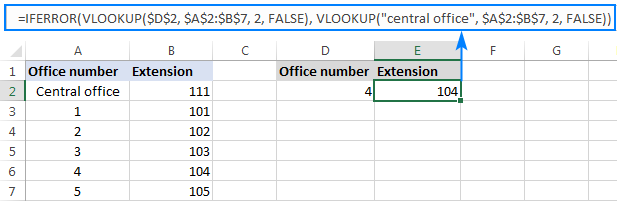If the office number is not found, the central office extension is displayed: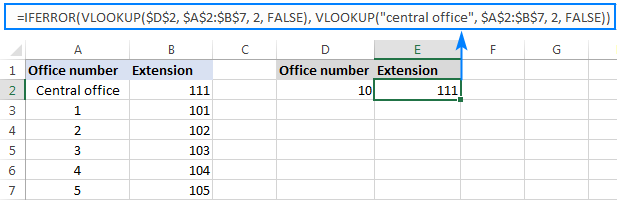To make the formula a bit more compact, you can use a different approach:

First, check if the number in D2 is present in the lookup column (please notice that we set col_index_num to 1 for the formula to look up and return value from column A): VLOOKUP(D2,\$A\$2:\$B\$7,1,FALSE)

If the specified office number is not found, then we search for the string "central office", which is definitely in the lookup list. For this, you wrap the first VLOOKUP in IFERROR and nest this whole combination inside another VLOOKUP function:

`=VLOOKUP(IFERROR(VLOOKUP(D2,\$A\$2:\$B\$7,1,FALSE),"central office"),\$A\$2:\$B\$7,2)`

Well, a slightly different formula, the same result: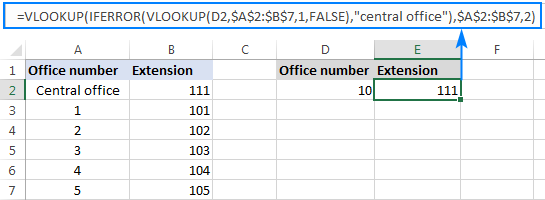But what is the reason to look up "central office", you may ask me. Why not supply the extension number directly in IFERROR? Because the extension may change at some point in the future. If this happens, you will have to update your data just once in the source table, without worrying about updating each of your VLOOKUP formulas.

## How to do sequential VLOOKUPs in Excel

In situations when you need to perform the so-called sequential or chained Vlookups in Excel depending on whether a prior lookup succeeded or failed, nest two or more IFERROR functions to run your Vlookups one by one:

The formula works with the following logic:

If the first VLOOKUP does not find anything, the first IFERROR traps an error and runs another VLOOKUP. If the second VLOOKUP fails, the second IFERROR catches an error and runs the third VLOOKUP, and so on. If all Vlookups stumble, the last IFERROR returns your message.

This nested IFERROR formula is especially useful when you have to Vlookup across multiple sheets as shown in the below example.

Let's say, you have three lists of homogeneous data in three different worksheets (office numbers in this example), and you want to get an extension for a certain number.

Assuming the lookup value is in cell A2 in the current sheet, and the lookup range is A2:B5 in 3 different worksheets (North, South and West), the following formula works a treat:

`=IFERROR(VLOOKUP(A2,North!\$A\$2:\$B\$5,2,FALSE), IFERROR(VLOOKUP(A2,South!\$A\$2:\$B\$5,2,FALSE), IFERROR(VLOOKUP(A2,West!\$A\$2:\$B\$5,2,FALSE),"Not found")))`

So, our "chained Vlookups" formula searches in three different sheets in the order we nested them in the formula, and brings the first match it finds: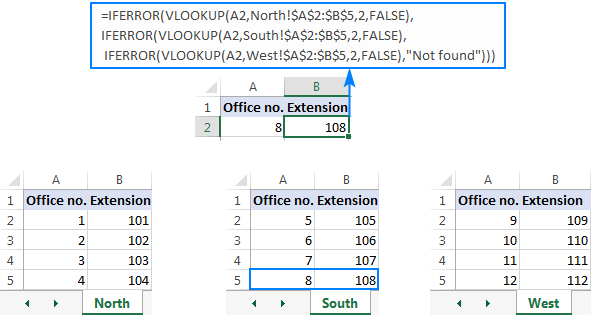You can find more examples in this article: VLOOKUP in multiple sheets in Excel.

This is how you use IFERROR with VLOOKUP in Excel. I thank you for reading and hope to see you on our blog next week!

## You may also be interested in

1. Hi Sir.
This formula is giving me "false" in result . Kindly help.
=IF(Q14>0.99,(IFERROR(VLOOKUP(Q14,Sheet3!\$G\$8:\$H\$144,2,0),"")))
Thanks

Sahil

• Hi! Your IF formula is missing the [value_if_false] argument. Try this formula:

=IF(Q14>0.99,IFERROR(VLOOKUP(Q14,Sheet3!\$G\$8:\$H\$144,2,0),""),"")

• It worked . Thanks a lot

2. I want to do a lookup from a different sheet and add the data of multiple cells in sheet 1, suppose, sheet 1 has this entry, 201, now i want to look it up in sheet 2, and need to add all values are in front of it in the sheet one. Suppose, sheet 2, Column A has 201, column B , 22, Column c, 44, Column D 155.. I want to put all values at the same time in sheet one .

• Hi! Use the INDEX MATCH function to search for a value. To get a row from a range using the INDEX function, read this article. For example,

=INDEX(B2:D10,MATCH(201,A2:A10,0),)

3. I am trying to duplicate the sequential VLOOKUP example but mine is not working.

=IFERROR(VLOOKUP(D10,CAR_CAP_MRC!\$A:\$K,2,0),IFERROR(VLOOKUP(D10,CAR_CAP_MRC!\$A:\$L,3,0),IFERROR(VLOOKUP(D10,CAR_CAP_MRC!\$A:\$L,4,0),IFERROR(VLOOKUP(D10,CAR_CAP_MRC!\$A:\$L,5,0),IFERROR(VLOOKUP(D10,CAR_CAP_MRC!\$A:\$L,6,0),"")))))

I have pulled the individual VLOOKUPS out separately to test them and they are all working perfectly with this part of the formula VLOOKUP(D10,CAR_CAP_MRC!\$A:\$L,4,0) resulting in the correct output but when I put them all together, I get no result.

• Hi! Unfortunately, I can't verify your formula as I don't have your data. Note that you use \$A:\$K in the first VLOOKUP and then \$A:\$L after that.

4. Hi,

I want pull data from Multiple Tabs using formula .

Example.

TAB1 - Name - Address - Phone number
Hari - BNG - 66666

TAB2 - Name - Address - Phone number
Anand-BNG-7777

5. i have two sheets where if the heading of a column matches with the value in another sheet q column then vlook up is executed. if it is false the it will jump to next heading this sequence goes on for 8 times .

but in third row though it is matching with the heading the we lookup formula is identifing a273 as 103642 where as it is 066-50007-1531

=IFERROR(IF(ac_matched!\$B\$1=removals_only!Q273,VLOOKUP(removals_only!A273,ac_matched!\$J:\$J,1,0),IF(ac_matched!\$C\$1=removals_only!Q273,VLOOKUP(removals_only!A273,ac_matched!\$K:\$K,1,0),IF(ac_matched!\$D\$1=removals_only!Q273,VLOOKUP(ac_matched!A273,ac_matched!\$L:\$L,1,0),IF(ac_matched!\$E\$1=removals_only!Q273,VLOOKUP(removals_only!A273,ac_matched!\$M:\$M,1,0),IF(ac_matched!\$F\$1=removals_only!Q273,VLOOKUP(removals_only!A273,ac_matched!\$N:\$N,1,0),IF(ac_matched!\$G\$1=removals_only!Q273,VLOOKUP(removals_only!A273,ac_matched!\$O:\$O,1,0),IF(ac_matched!\$H\$1=removals_only!Q273,VLOOKUP(removals_only!A273,ac_matched!\$P:\$P,1,0),IF(ac_matched!\$I\$1=removals_only!Q273,VLOOKUP(removals_only!A273,ac_matched!\$Q:\$Q,1,0),"")))))))),"nhpn")

6. Hi -

Thank you for your valuable help.

This question is closely, though not perfectly, aligned with this topic.

I frequently have the pattern
=if( iserror (vlookup(...),"error", vlookup(...))
where the arguments to the two vlookups are identical. And where it's very easy to get the two argument lists out of sync.

If there a way to avoid the duplication?

To help illustrate... in programming languages I could do something like
x = vlookup(...)
if iserror(x) return "error"
else return x

Thanks,
Mike

7. Hi
Thanks a lot for your help, i want a formula based on the following thanks

=IFERROR(VLOOKUP(C452,sheet1!\$C\$1:\$S\$100,7,FALSE),
IFERROR(VLOOKUP(C452,sheet2!\$C\$1:\$R\$100,7,FALSE)))

if 7 COLUMN has two values first 0 and second above then 0
i want vlookup to skip the 0 and get the second value

7 = 0
7 = 1

• Hello!

8. Hi!

I had a problem with my IFERROR VLOOKUP formula. It does work if I input a value on the search key but when I used IMPORTRANGE formula on the search key, the IFERROR VLOOKUP formula gives a blank cell.

Can you help me with this.

Thank you.

9. I have a following fx that looks up data on two tables to return a value depending on the audience group. This is the current fx that returns the correct results: =IF(VLOOKUP('Master Role-Course Data'!\$A\$1,'Master Role-Course Data'!\$A\$3:\$AB\$43,H9)=0,"",VLOOKUP('Master Role-Course Data'!\$A\$1,'Master Role-Course Data'!\$A\$3:\$AB\$43,H9)).

However, I want to add an 'if error' to add text prompting the user to perform an action to select a business group in another cell otherwise the return cell will show the standard "N/A" value.

• Hi!

10. Hello, great info!
I was wondering what a formula would look like to display the difference between 2 cells, not just "match" or "not match", example below.

cell A1 contains the following text - pie,cake,donut.

cell B1 contains the following text - pie,cake,donut,taco.

in cell C1 we would like to see output = taco

11. Is there a way to add another criteria to this formula? I want to add if this range has a certain word, in my case OUTSTANDING, to return BLANK"". Current Formula: IFERROR(VLOOKUP(B9,'REPORT!A:C,3,FALSE),"")

• Hello!
Based on your description, it is hard to completely understand your task. However, I’ll try to guess and offer you the following formula with IF statement:

=IF(SUM(--(A:C="OUTSTANDING")),"",IFERROR(VLOOKUP(B9,A:C,3,FALSE),""))

12. Hi , just want to ask how to called one raw one data and make exceptional towards data not to call in and remarks as NA . For example , i got Malaysia and London in one row of data and try to called out for malaysia only and make the data of london come out as NA

• Hi!
I am not sure I fully understand what you mean. Here is the article that may be helpful to you: Fixing #N/A error in VLOOKUP. If this is not what you wanted, please describe the problem in more detail.

13. Hello, can you kindly help me understand what we can use this formula for?

=IFERROR(VLOOKUP(A17381,A:AA,25,FALSE)-VLOOKUP(IF(J17381=1,I17381)&0&(((MONTH(DATE(I17381,J17381,1)-1)))&0)&(K17381&0)&L17381,A:AA,25,FALSE),0)

• Hi!
It is very difficult to understand a formula that contains unique references to your workbook worksheets. What is the problem you want to solve?

• I am trying to do a computation where Jan will be subtracted from Feb, March from Feb without having to manually change the months

=IFERROR(VLOOKUP(A17381,A:AA,25,FALSE)-VLOOKUP(IF(J17381=1,I17381)&0&(((MONTH(DATE(I17381,J17381,1)-1)))&0)&(K17381&0)&L17381,A:AA,25,FALSE),0)

=IFERROR(VLOOKUP(A17381,A:AA,25,FALSE)-VLOOKUP((I17381&0)&(((MONTH(DATE(I17381,J17381,1)-1)))&0)&(K17381&0)&L17381,A:AA,25,FALSE),0)

The second formula worked all the months from 2018 to 2021 but its not able to perform same subtraction in 2022 from Jan to Feb

• Hi!
The information you provided is not enough to understand your case and give you any advice, sorry. What does the phrase mean "Jan will be subtracted from Feb"? Could you please describe it in more detail? What result do you want to get? Give an example of the source data and the expected result.

14. Hi,
I came across your solution to a poster's problem posted in the comments on Nov 30th.

=IF(IFERROR(VLOOKUP(E2,A1:B9,2,FALSE),"")="","", IFERROR(VLOOKUP(E2,A1:B9,2,FALSE),""))

This to me seems to be a neat solution to dealing with the problem when VLOOKUP returns #N/A or 0.

I'm struggling to understand how it works. As I read it it works as follows but it doesn't make sense

If VLOOKUP outputs an error, write "" as output and if output is "" then write "".

If vlookup does not output an error (i.e. a result or 0) then run vlookup again.

So the first IfError traps #N/A as "" but I don't see how the second IfError differentiates between a result and 0.

Thank you.

15. Loved the simplicity

16. HI I WANT TO FIND OUT ONLY ONE PARTICULAR PRODUCT OUT OF THREE PRODUCTS BY MATCHING

FOR EXAMPLE: THERE ARE DIFFERENT VALUES FOR KIRAN OUTLET LIKE BISCUIT-CHOCALATE-DRINK AND OUT OF THEM IF I WANT TO IDENTIFY ONLY BISCUIT HOW TO FIND OUT

• Hi!
To find subtext within text, you can use the formula

=ISNUMBER(SEARCH("BISCUIT",A1,1))

If this is not what you wanted, please describe the problem in more detail.

17. hi
kiran enterprises has three different products,

for example I want to match product wise and give value

result should be like this - outlet name - biscuits - chocalate - drink

outlet name biscuits drink chocalate
kiran 25 35 65

18. Hi, this works! I learnt a lot. Thanks.
I am not a native English speaker, but I think your example and words are easy to understand.
Thanks again.

19. Alexander who are you, man ??......I really don't have words to thank you.

My requirement was too much complex and too much ifs and what ifs and only you did it.( I contacted other guys to make this formula)
Now this formula is perfect.

Last but not the least... so much apologies for wasting your time and effort by telling the info in bits and pieces ( I keep on smiling while thinking about this) And loads of thanks and good wishes for you.

20. how to make a formula for first looking up a value in one sheet(sheet A) and if not found then look that value in another sheet(sheet B) and after finding that value in sheet B, returns the message rather than returns the sheet B value.

• Hi,
I think the formula might look something like this:

Here is the article that may be helpful to you: VLOOKUP with IF statement in Excel.

• OooH...Love u Alexander. The formula worked.
IS there anything I can do for u?

• Hi!
Thank you for your positive feedback! It’s the best incentive for us to keep up and improve :)

• Hi!
Hope u will be in good health.
Can I let myself clear on the syntax of the following formula u made for me.

Some of my understanding: If I start from inside out;

ISERROR Syntax; Looking up B2 in table1,if an error(e.g#NA) returns then it is true, else false.

IF syntax ; if ISERROR returns 'true'(i.e an error), it would write 'Not Found' and if ISERROR returns false (i.e no error) then it would return text 'Found' .
Here 'ISERROR' is logical text of 'IF' function.

There must be some error (i.e #NA) to be traped by ISERROR in order to return the text "Not Found" or ''Found''.

But if I am correct ( definitely not), the result of formula is inconsistent with the above understanding.
Your formula works like: if the value (B2) is in 'Lookup table', it returns that value and if it does not find B2,it goes to 'Lookup table1' and if B2 is found ,it provides text 'Found' and if it is not found in 'Lookup table1' either then it says 'Not Found'.

• Hello!
I wrote this formula based on the description you provided in your original comment. You didn't say anything about what to do if a value is found in table A. Therefore, a value is returned.
I didn't quite understand what you want. Perhaps a lookup in the first table should also return a message.

• Ok Alexander I make myself simple.

First, I want to understand the syntax of the formula.

Second, your formula works very well. It returns the value of Table A and if not found here, it finds the value in Table B and if it finds the value in Table B, returns the message instead of the value. If the value is not found in both the tables, it returns ''Not Found but if there is NILL value, it also returns Not found.

I want that this formula would return nothing if I give nothing in the cell.

• Hi,
Can you fully describe your problem? I waste a lot of time redoing your formula.
"if I give nothing in the cell" — what cell are you talking about now? About B2? About \$A\$2:\$B\$5? Or all of them?

• Apologies for not being clear Alexander and so much apologies for wasting your precious time.

If I let B2 empty, the formula would return nothing.

• Hello!
The formula below will do the trick for you:

Hope this is what you need.

• Oh great great Alexander. It worked.

Your response time is so amazing. I am really unable to understand how you manage to do so. You have helped me so much in my job.

• Hi,

Alexander one thing was missing.

The value of A!\$A\$2:\$B\$5 is not able to return. Instead the text string (Found) has returned.

Apologies. May I send you the data if you are comfortable with?

• Hi,
Try the following formula:

Hope you haven't forgotten anything else.

21. Hi

I am hoping that you can help me... I am struggling a lot with creating a spreadsheet of data on students marks and obtaining their grade depending on what level test they completed. So...:

Tab 1 - student data with marks:
Mark column 1, standard/ higher test, grade (what I am trying to find out)

Tab 2 - marks and grades
Table 1 - standard test
Mark column 1, grade column 2

Table 2 - higher test
Mark column 1, grade column 2

I require the mark to be obtained from tab 1, along with the column stating either standard or higher test - then obtaining the correct grade from tab 2.

I am hoping you can help me as I have searched everywhere and although I have got an IFERROR function and VLOOKUP I cannot determine how to look up 2 values (mark and test type) and then look at 2 different tables to determine which grade is correct.

So far I have this:

=IFERROR(LOOKUP(D4,'CP7-8'!\$A\$3:\$B\$37),0)

But that is just for looking up 1 value and 1 table???

• Hello!
Please check out the following article on our blog, it’ll be sure to help you with your task: Vlookup multiple matches in Excel with one or more criteria

22. I am trying to make a formula to check multiple workbooks, I want the formula to check if an item is in column A e.g an egg then check to see if there is an amount in column C e.g 3, if not move on to another workbook to check the same columns. If there is an amount over 1, I want it to say yes and if it is 0 to say no.

• Hello!
Sorry, I do not fully understand the task. If you only use one workbook then the formula is -

=IF(AND(A1="egg",C1>3),"Yes","No")

If this is not what you need, please explain your problem in more detail. Give an example of the source data and the expected result.

23. Hello,

I am trying to do a vlookup function while returning value as below:

1. if blank cell, it will return blank
3. if there's a value, it will return the value.

Currently my formula is like this -

=IF(IFERROR(VLOOKUP(E2,A1:B9,2,FALSE),FALSE),(VLOOKUP(E2,A1:B9,2,FALSE)),"")

This formula works only if the value is integer but I encounter an error if the value inside the cell is a string. It will return as this - #VALUE for a string value.

• Hello!
If I understand your task correctly, the following formula should work for you:

=IF(IFERROR(VLOOKUP(E2,A1:B9,2,FALSE),"")="","", IFERROR(VLOOKUP(E2,A1:B9,2,FALSE),""))

Hope this is what you need.

24. I am trying to combine two If statements with their own VLookup. I can get it to work if its just one, but not the other. I am using an IF statement to return the data based on the value of cell X, e.g. if cell x = ND, Vlookup to return the value, and if cell x = ED, Vlook up to return the value.

=IF(I6="ND",VLOOKUP(J6,'Post Codes'!A2:B117,2,FALSE),"", IF(I6="ED",VLOOKUP(J6,'Post Codes'!A2:E117,5,False)""))

25. Is there a way to get from which sheet was the value picked up, for example in this case its south, and if there are multiple can it display from what sheets, the value was picked up.

• Hello!
To see how your formula is being executed, you can use the Formula - Evaluate Formula menu item.

26. This is fantastic stuff, but I have an issue that I cannot seem to get around.. Can you pls pls pls help..

=IFERROR(VLOOKUP(\$N3245,'GB30'!\$A\$2:\$K\$99999,3,FALSE),IFERROR(VLOOKUP(\$N3245,'GB03'!\$A\$2:\$G\$9999,4,FALSE),"DNR"))))))

Looking in 2 sheets for a given value and returning the associated lookup value when it's there. This is working fine, but when a cell is blank I am getting a "Zero" in the result. But I want it to show as an "empty cell" or "". How can these be adapted to result in ""

I have been stuck on this for days!.

Jason

• Hello!
You can check the obtained values using the IF function:

=IF(IFERROR(VLOOKUP(\$N3245,’GB30′!\$A\$2:\$K\$99999,3,FALSE),IFERROR(VLOOKUP(\$N3245,’GB03′!\$A\$2:\$G\$9999,4,FALSE),”DNR”))))))=0,"",IFERROR(VLOOKUP(\$N3245,’GB30′!\$A\$2:\$K\$99999,3,FALSE),IFERROR(VLOOKUP(\$N3245,’GB03′!\$A\$2:\$G\$9999,4,FALSE),”DNR”)))))))

27. Having trouble with the correct IF and VLookup formula. I have 2 separate sheets; 1 with names and the second with names and email addresses. I need a formula that will check to see if the a name on the first sheet is on the second sheet, and if it is, bring over the email address to the first sheet, in a new cell. Any ideas?

28. Mine worked Perfectly!!!!!!!
Thank you so much for the explanation, very easy to follow. :)

29. Hi!
I am trying to have my formula look up a text value and if the text is not present then move to the next row under it. If the text is present I want it populate onto a new sheet, if it isn't present then I don't want it to appear at all and I don't want blank rows to populate. Please help. Thanks in advance!

What you refer in the first sentence can be done only with the help of VBA.
What you mention further, unfortunately I haven't got what you mean.

30. =IFERROR(VLOOKUP(A2,'Balance'!A:C,2,FALSE),0) is not working when A2 more than A999 how to solve it

• Hello!
I have checked the work of your VLOOKUP function in my own file and haven’t found any error. However, the formula I used looks as follows:

=IFERROR(VLOOKUP(A2,[’Balance’]Sheet1!A:C,2,FALSE),0)

May this be the problem? If you are still getting an error, please describe the problem in more detail so that I’ll be able to help you better.

31. when we revise the VLOOKUP Function, what is replaced with COLUMN Function

32. I'm using excel office 360. When I do the vlookup, am getting the return value of the "text formula I typed in". How to fix this?

33. Hello Mam,
Please send me a video of IFERROR formula with vlookup.

Regard,

35. you are my number one !!!

36. Please send me main excel accounting formulas at above email

37. Hi, mam
I have a data in sheet first is name and second is dob i want to do if month is coming dob highlights automatically

38. Column A2:A173 contains 1,2 or is blank
column c contains a date or is blank

I need a formula to do the following:
If column a contains a 1 or 2 look at the same row in column C and if that contains ANY entry (will most likely be a date) count it.

39. Your timing is excellent. Just started new job and they gave me 3 files with 1 million rows each. Of couse split amongst tabs. I was going crazy with all the vlookup and now you helped me save hours of work. Thank you thank you.

40. Hi

Is there a way i can get a cell to return a blank cell after a particular date? if its before a date then use zero. Im having trouble with so many commands.